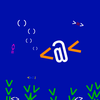# learning - Dhall

Welcome to the Functional Programming Zulip Chat Archive. You can join the chat here.I'm trying to wrap my head around dhall. I'm trying to create a function and I'm not entirely sure how I'm messing it up. Any tips please?
I'm trying to create this yaml

``````        - check: this.content == "/commands"
processors:
- bloblang: |
let commands = [
"/commands",
"/joke",
"/roast",
"/release",
]
root = "My commands are: " + \$commands.join(", ")
``````

With this dhall

``````let processorWithArgs =
\(checkArg: Text) ->
\(args: List) ->
\(message: Text) ->
{ check = "this.content == \"/\${checkArg}\""
, processors =
{ bloblang = "let \$checkArg = \${args}"
, root = message
}
}

in [ processorWithArgs "commands"
, [ "/commands",
"/joke",
"/roast",
"/release",
]
, "My commands are: \" + \$commands.join(\", \")" ]
``````

But I'm getting this output

``````Error: Invalid function input

3│     \(args: List) ->
4│     \(message: Text) ->
5│     { check = "this.content == \"/\${checkArg}\""
6│     , processors =
7│     { bloblang = "let \$checkArg = \${args}"
8│     , root = message
9│     }
10│     }
11│

processor.dhall:3:5
``````Its looking like it doesn't like the `List` time for `args`. I guess I need to figure out how to map a list to the TextSo right now i'm at

``````let processorWithArgs =
\(checkArg: Text) ->
\(args: Text) ->
\(message: Text) ->
{
check = "this.content == \"/\${checkArg}\""
, processors = {
bloblang = "let \${checkArg} = \${args}"
, root = message
}
}

in [ processorWithArgs "commands"  "\"/commands\", \"/joke\", \"/roast\", \"/release\","  "My commands are: \" + \$commands.join(\", \")" ]
``````

And that gets me

``````- check: "this.content == \"/commands\""
processors:
bloblang: "let commands = \"/commands\", \"/joke\", \"/roast\", \"/release\","
root: "My commands are: \" + \$commands.join(\", \")"
``````

which is close. But its putting the escaped quotes in the yamlSo to start with, I was reading my yaml wrong. bloblang on down is one big text field

``````let processorWithArgs =
\(checkArg: Text) ->
\(message: Text) ->
{
check = "this.content == \"/\${checkArg}\""
, processors = {
bloblang = "let \${checkArg} = \${message}"
}
}

in [ processorWithArgs "commands"  ''
let commands = [
"/commands",
"/joke",
"/roast",
"/release",
]
root = "My commands are: " + \$commands.join(", ")''
]
``````

gives me

``````- check: "this.content == \"/commands\""
processors:
bloblang: |
let commands = let commands = [
"/commands",
"/joke",
"/roast",
"/release",
]
root = "My commands are: " + \$commands.join(", ")
``````

So now I just need to understand how not to return those escaped quotes on the first line.I guess it sanitizes your output on purpose to make it safe
May it be that the resulting YAML will still work though?